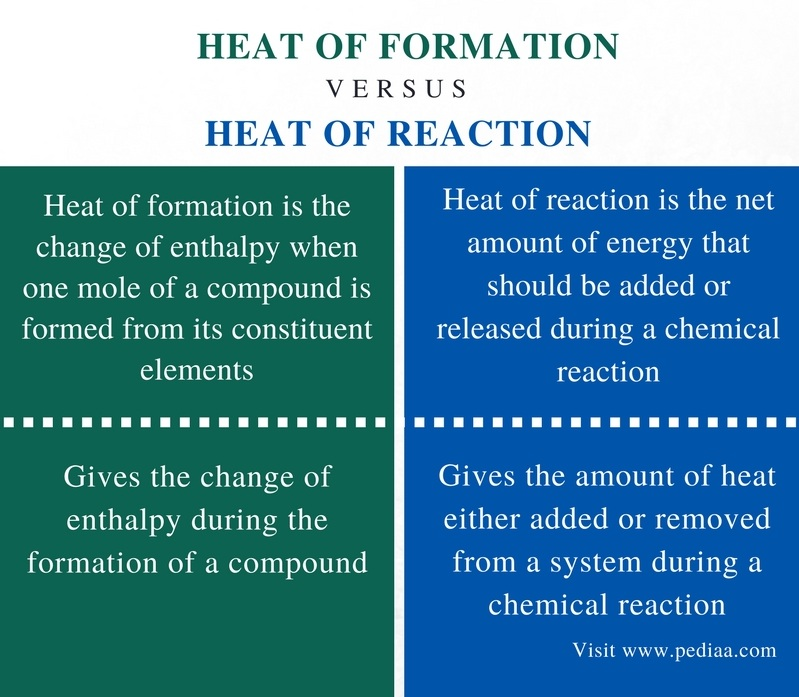# Difference Between Heat of Formation and Heat of Reaction

## Main Difference – Heat of Formation vs Heat of Reaction

Enthalpy is the heat energy that is being absorbed or evolved during the progression of a chemical reaction. The enthalpy is given the symbol H. H indicates the amount of energy. The change of enthalpy is given as ∆H, and the symbol ∆ indicates the change of enthalpy. The heat of formation and heat of reaction are two forms of enthalpies. The main difference between heat of formation and heat of reaction is that heat of formation is the amount of energy either absorbed or released during the formation of a compound whereas heat of reaction is the amount of energy either absorbed or released during any chemical reaction.

### Key Areas Covered

1. What is Heat of Formation
– Definition, Equation
2. What is Heat of Reaction
– Definition, Equation
3. What is the Difference Between Heat of Formation and Heat of Reaction
– Comparison of Key Differences

Key Terms: Endothermic, Enthalpy, Exothermic, Heat, Heat of Formation, Heat of Reaction## What is Heat of Formation

The heat of formation is the change of enthalpy when one mole of a compound is formed from its constituent elements. This is called standard heat of formation when it is given for the formation of substances at their standard states. The standard pressure is 105 Pa (1 bar), as recommended by IUPAC. However, there is no defined standard temperature. Hence, the heat of formation is given for a constant pressure. The symbol for the standard heat of formation is ΔHf°. It can be calculated using the following equation.According to this equation, the standard heat of formation is equal to the difference between the sum of the standard enthalpies of formation of the product and the standard enthalpies of formation of reactants.

## What is Heat of Reaction

The heat of reaction is the net amount of energy that should be added or released during a chemical reaction. In other words, it is the change in enthalpy in a chemical reaction that occurs at a constant pressure. The heat is either added or released in order to keep all the reactants at a constant temperature. It is measured per mole of a substance. The heat of reaction is given as below. When this is defined for the standard state, it is known as standard enthalpy of reaction. The standard state is the pure substance at 1 bar pressure and at a relevant temperature.If the value of the heat of reaction is a positive value, it indicates that the reaction is endothermic (in which the heat is absorbed from outside). But if the value of the heat of reaction is a negative value, it indicates that the reaction is exothermic (in which heat is given off).

## Difference Between Heat of Formation and Heat of Reaction

### Definition

Heat of Formation: Heat of formation is the change of enthalpy when one mole of a compound is formed from its constituent elements.

Heat of Reaction: Heat of reaction is the net amount of energy that should be added or released during a chemical reaction.

### Concept

Heat of Formation: Heat of formation gives the change of enthalpy during the formation of a compound.

Heat of Reaction: Heat of reaction gives the amount of heat either added or removed from a system during a chemical reaction.

### Conclusion

Heat of formation and heat of reaction are two types of enthalpies defined for chemical reactions. The main difference between heat of formation and heat of reaction is that heat of formation is the amount of energy either absorbed or released during the formation of a compound whereas heat of reaction is the amount of energy either absorbed or released during any chemical reaction.

##### Reference:

1. “Standard Enthalpy of Formation.” Chemistry LibreTexts, Libretexts, 9 Feb. 2017, Available here.
2. “Heat of reaction.” Encyclopædia Britannica, Encyclopædia Britannica, inc., 4 Apr. 2016, Available here.
3. Helmenstine, Anne Marie. “Learn About Enthalpy Change from Heat of Formation Worked Problems.” ThoughtCo, Available here.